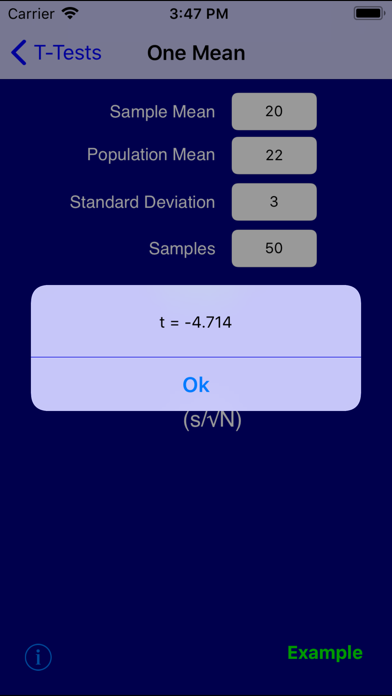Statistics Pro

iOS UniversalEducation

Free was 0.99

The reviews asked for it, you've got it! The linear regression and data analysis solvers now each handle 30 inputs!

Are you doing math with an abacus? No? Well, why are you using Z-score tables? Enter the mean and standard deviation of a bell curve, and this application computes the area under the curve to the left of any value you specify.

Or, compute the value on a bell curve given the area beneath it.

"Statistics Pro" contains a rich collection of solvers, examples, and tutorials for the following topics:

- Data analysis (mean, standard deviation, 1st quartile, median, 3rd quartile, and outlier thresholds for up to 30 data values)
- Linear regression (best-fit line and correlation coefficient on up to 30 points)
- Chebyshev's Theorem
- Normal Distribution
- Binomial Distribution
- Chi Squared Distribution
- Poisson Distribution
- Exponential Distribution
- Central Limit Theorem
- T-Tests for One and Two Means
- Confidence Intervals
- Uniform random number generation
- Binomial random number generation
- Gaussian random number generation
- Poisson random number generation
- Exponential random number generation

I welcome all comments, questions, and suggestions: lfeldman65@gmail.com

Follow me on facebook for app news, tips, and free Math help:

Please check out my other applications:

ACT Math Prep
Algebra Pro
Calculus Pro
Finite Math Pro
Geometry Pro
Math Pro (six Math apps for the price of two!)
Physics Pro
PreCalculus Pro
Probability Pro
SAT Math Prep

What's New

- Fixed bug that was preventing Normal Distribution examples from appearing

•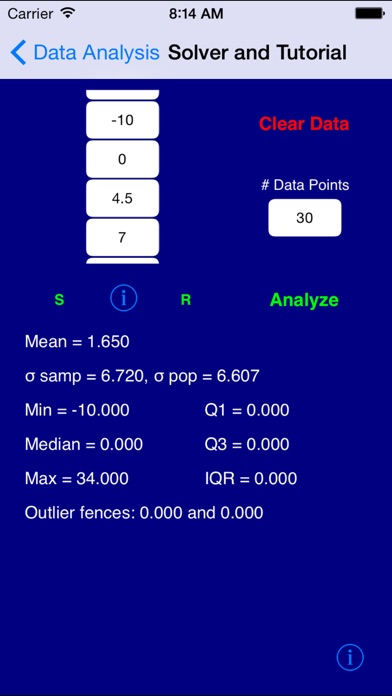•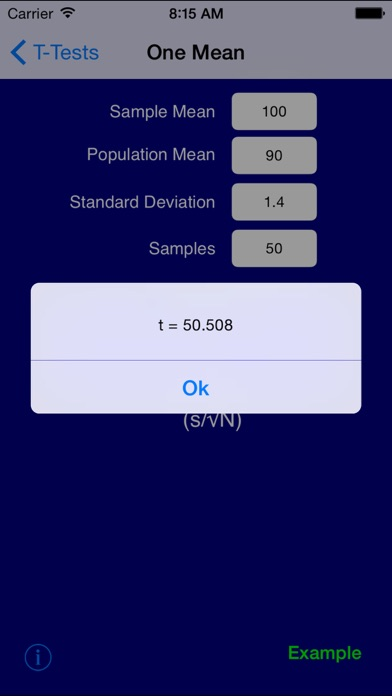••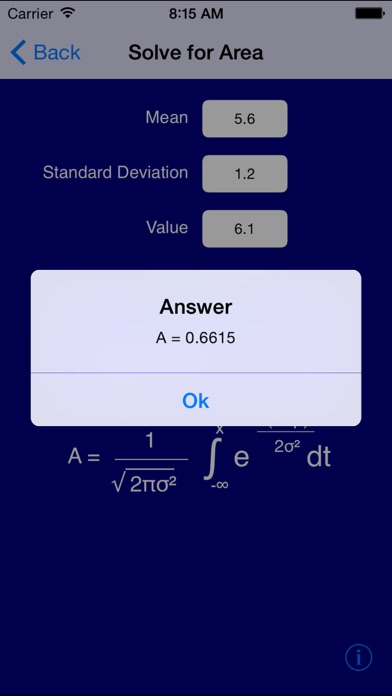•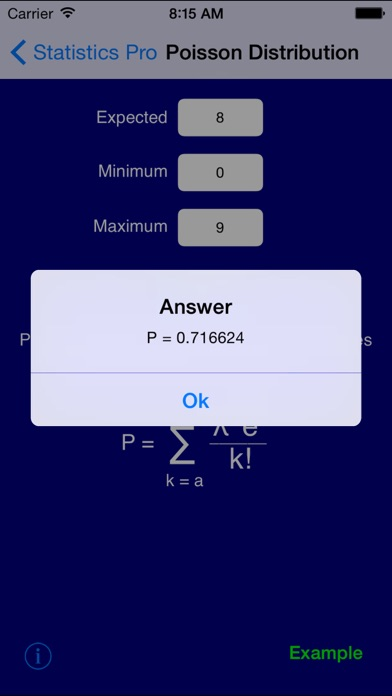•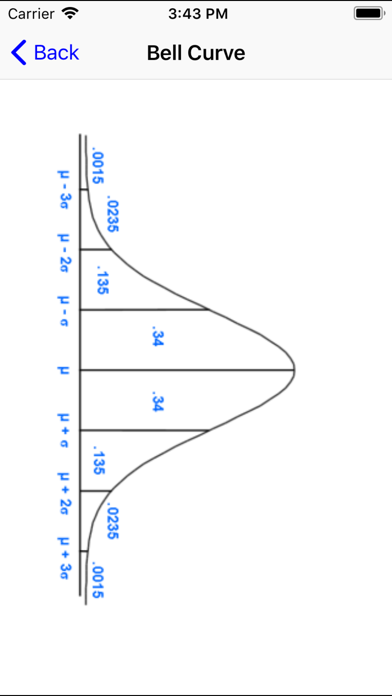•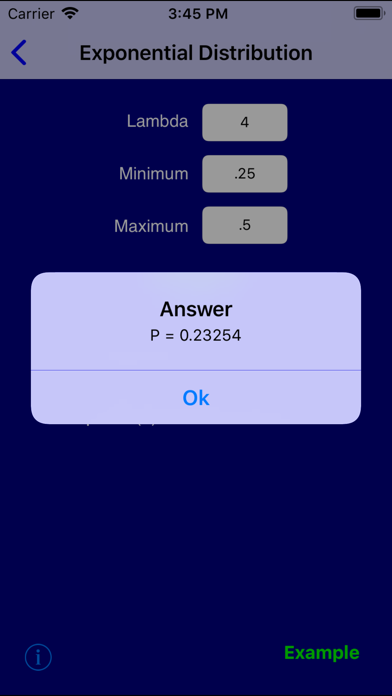•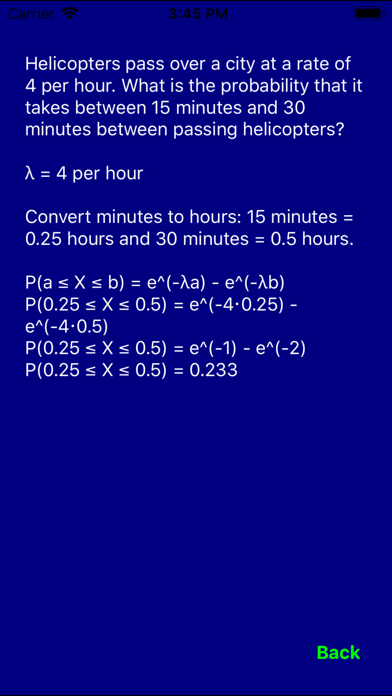•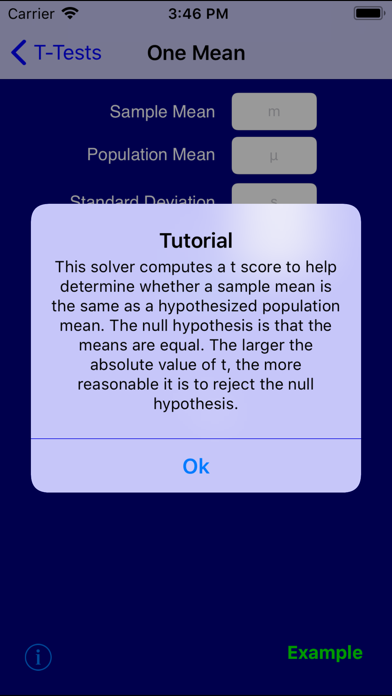•